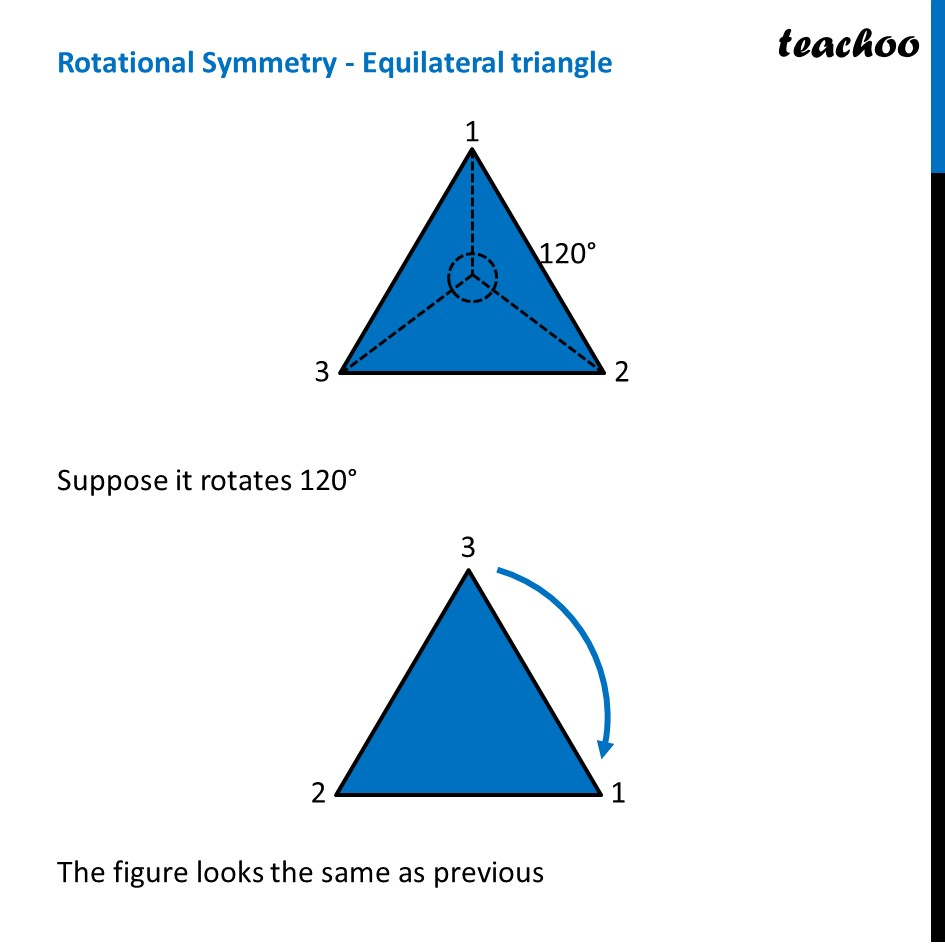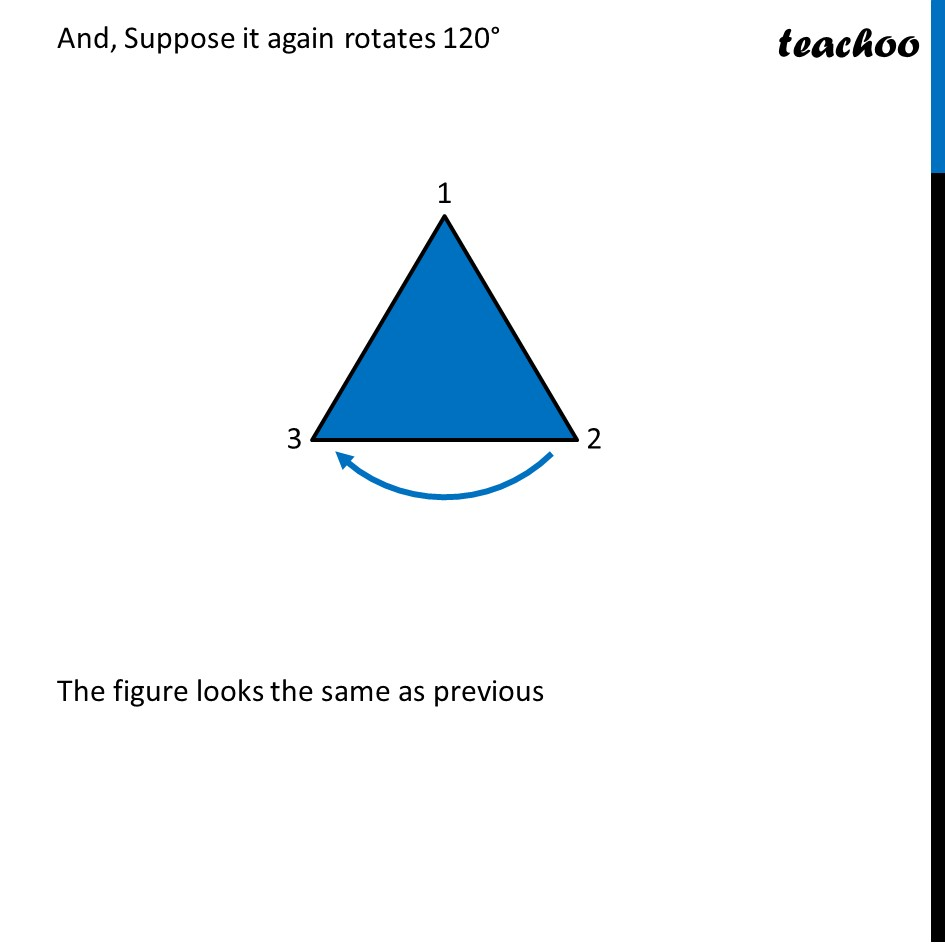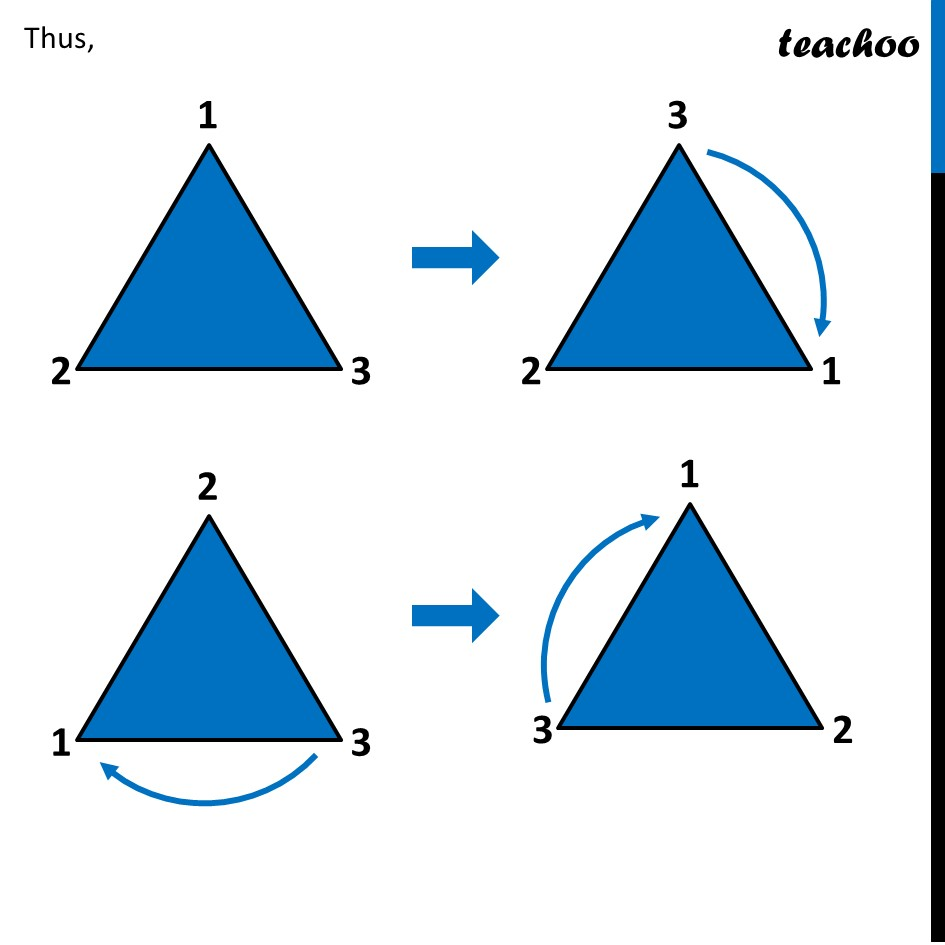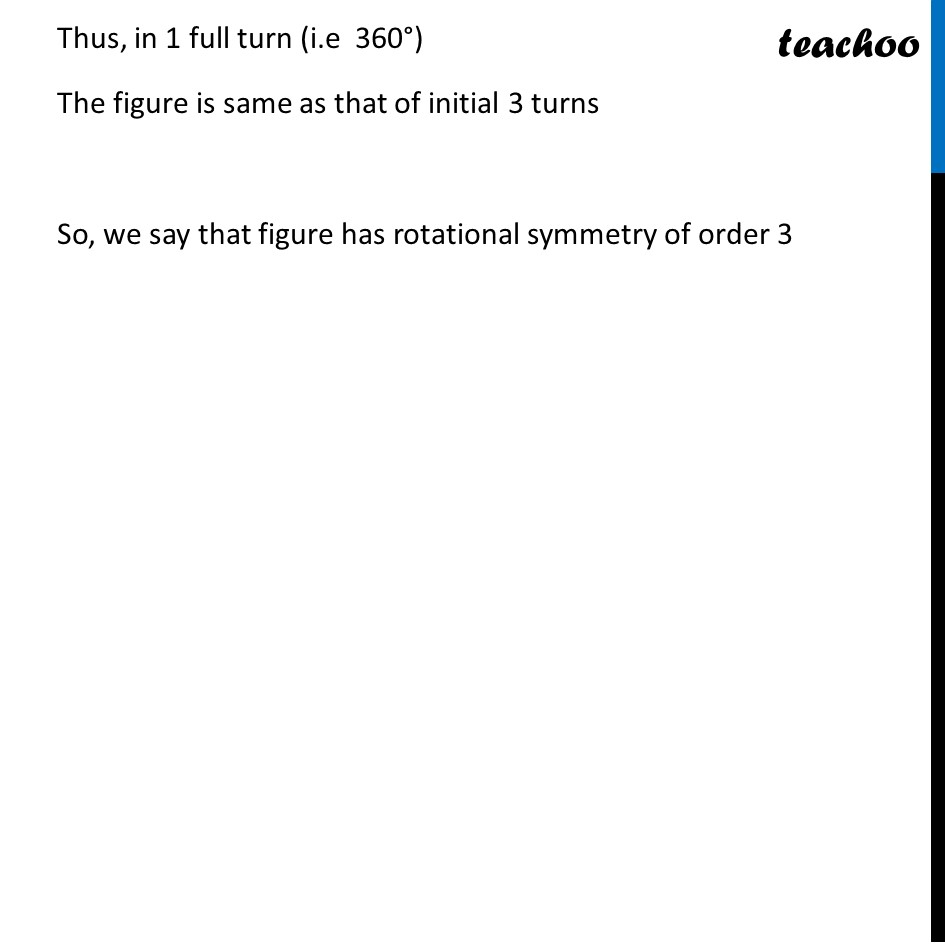Rotational Symmetry

Chapter 14 Class 7 Symmetry
Concept wise### Transcript

Rotational Symmetry - Equilateral triangleSuppose it rotates 120° The figure looks the same as previous And, Suppose it again rotates 120° The figure looks the same as previous Thus, Thus, in 1 full turn (i.e 360°) The figure is same as that of initial 3 turns So, we say that figure has rotational symmetry of order 3